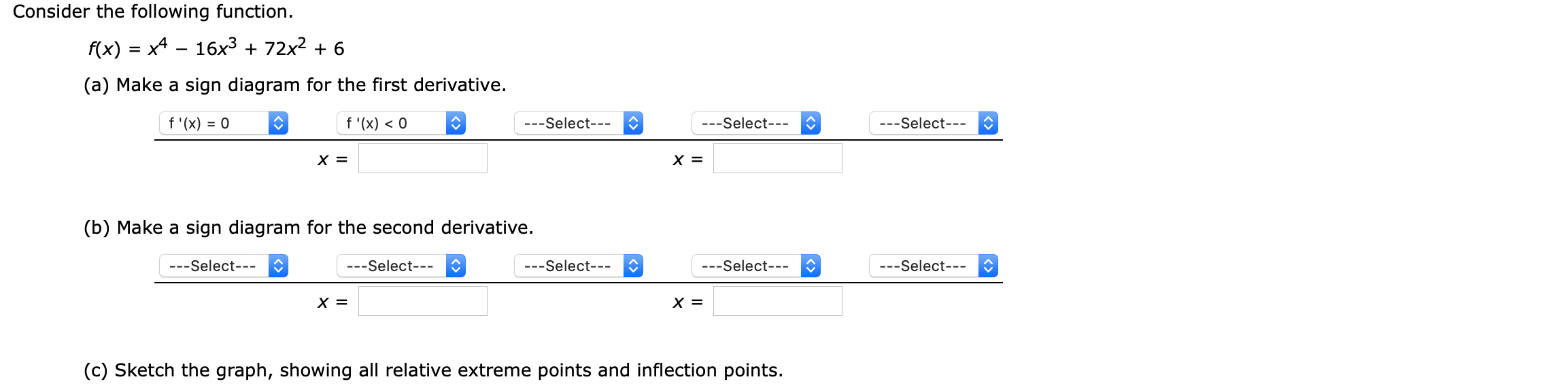# Question Consider the following function. f(x) = x4 – 16x3 + 72x2 + 6 (a) Make a sign diagram for the first derivative. f'(x) = 0 f'(x) < 0 ---Select--- ---Select--- ---Select--- X = X = (b) Make a sign diagram for the second derivative. ---Select--- ---Select--- ---Select--- ---Select--- ---Select--- X = X = (C) Sketch the graph, showing all relative extreme points and inflection points.